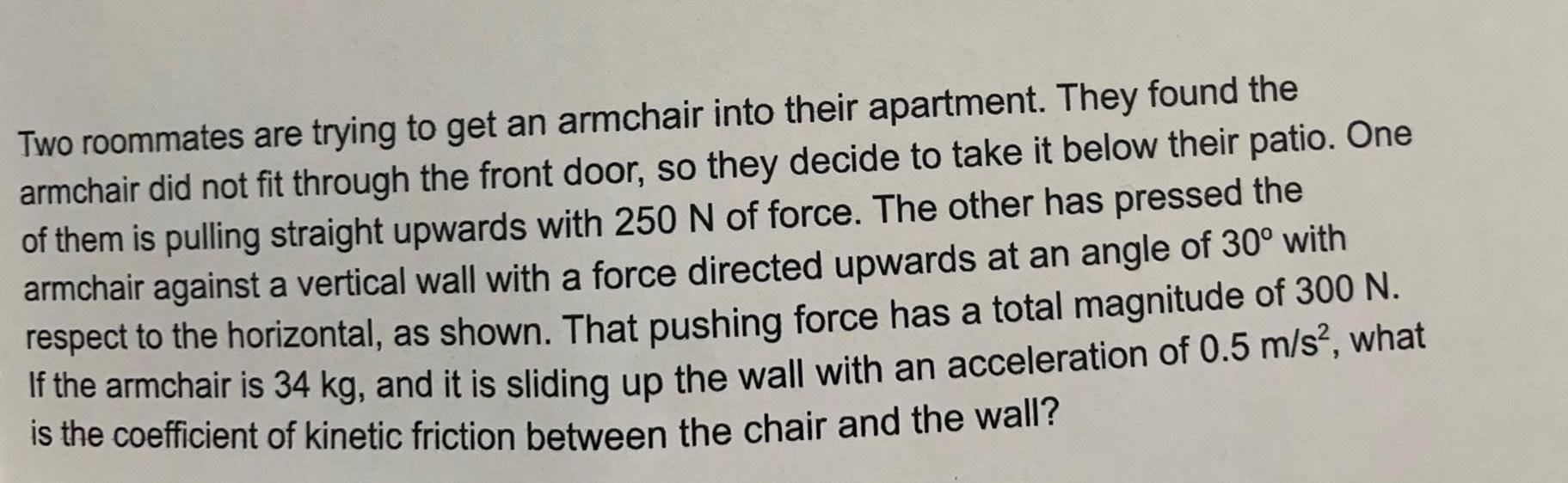Physics
Friction
Two roommates are trying to get an armchair into their apartment. They found the armchair did not fit through the front door, so they decide to take it below their patio. One of them is pulling straight upwards with 250 N of force. The other has pressed the armchair against a vertical wall with a force directed upwards at an angle of 30° with respect to the horizontal, as shown. That pushing force has a total magnitude of 300 N. If the armchair is 34 kg, and it is sliding up the wall with an acceleration of 0.5 m/s², what is the coefficient of kinetic friction between the chair and the wall?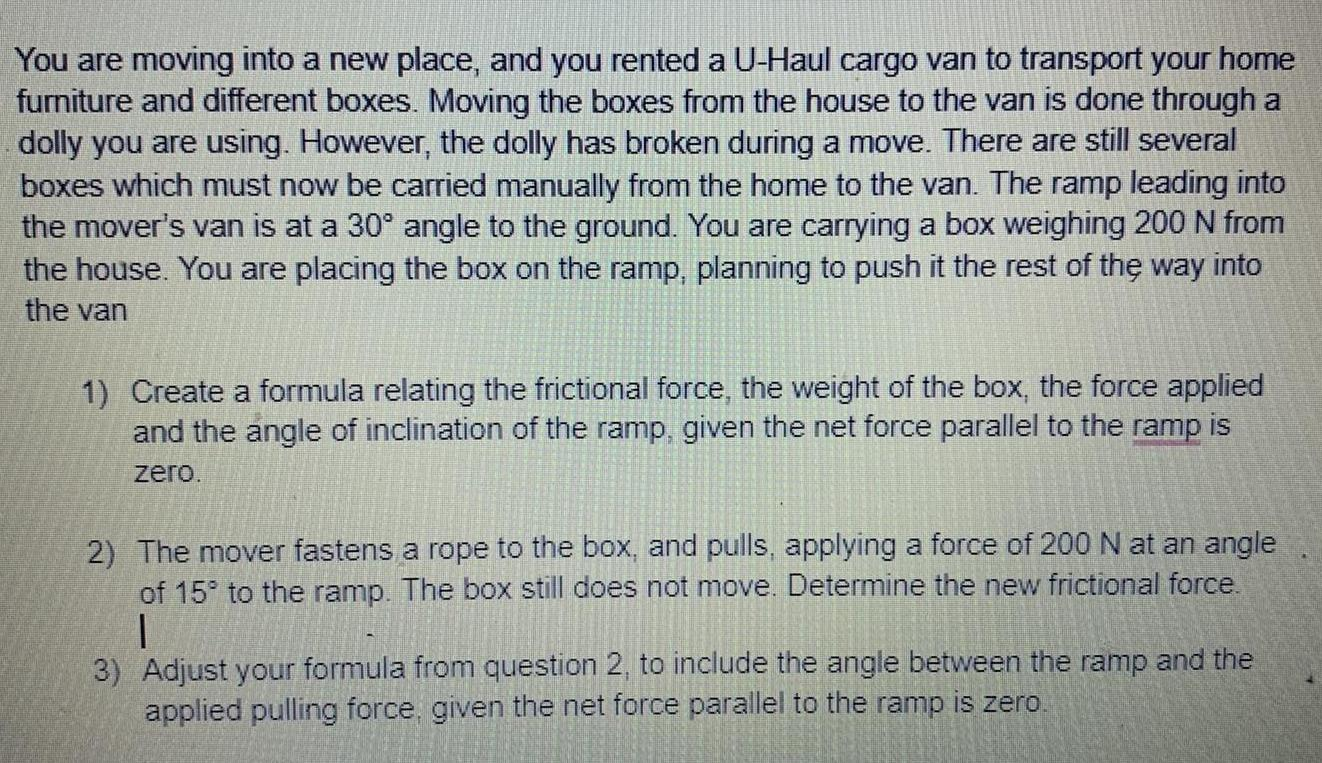Physics
Friction
You are moving into a new place, and you rented a U-Haul cargo van to transport your home furniture and different boxes. Moving the boxes from the house to the van is done through a dolly you are using. However, the dolly has broken during a move. There are still several boxes which must now be carried manually from the home to the van. The ramp leading into the mover's van is at a 30° angle to the ground. You are carrying a box weighing 200 N from the house. You are placing the box on the ramp, planning to push it the rest of the way into the van 1) Create a formula relating the frictional force, the weight of the box, the force applied and the angle of inclination of the ramp, given the net force parallel to the ramp is zero. 2) The mover fastens a rope to the box, and pulls, applying a force of 200 N at an angle of 15° to the ramp. The box still does not move. Determine the new frictional force. 3) Adjust your formula from question 2, to include the angle between the ramp and the applied pulling force, given the net force parallel to the ramp is zero.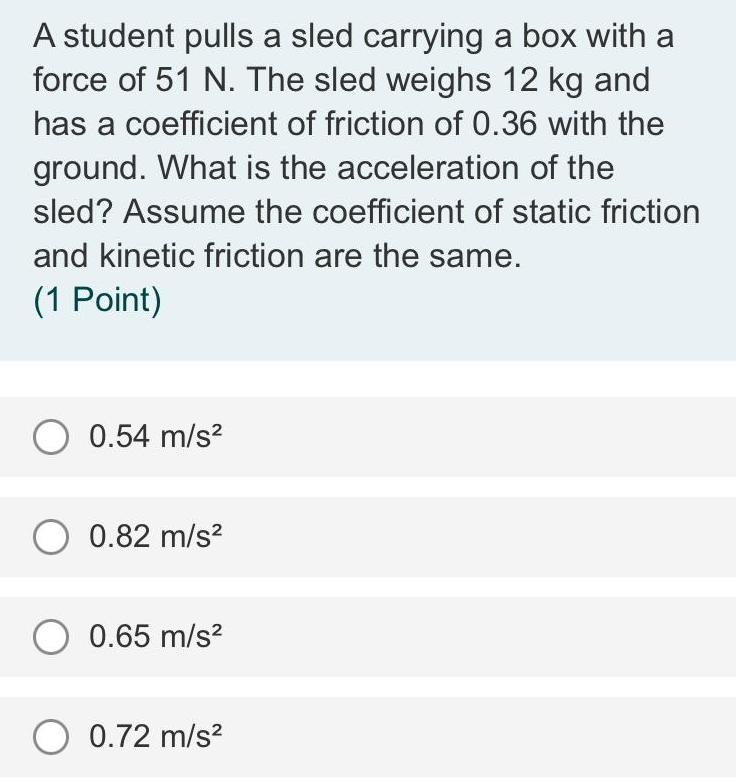Physics
Friction
A student pulls a sled carrying a box with a force of 51 N. The sled weighs 12 kg and has a coefficient of friction of 0.36 with the ground. What is the acceleration of the sled? Assume the coefficient of static friction and kinetic friction are the same. 0.54 m/s² 0.82 m/s² 0.65 m/s² 0.72 m/s²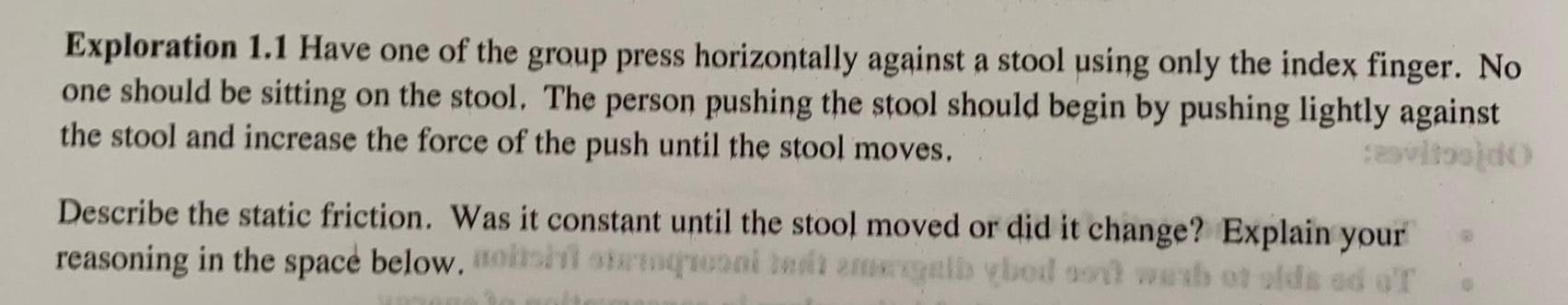Physics
Friction
Exploration 1.1 Have one of the group press horizontally against a stool using only the index finger. No one should be sitting on the stool. The person pushing the stool should begin by pushing lightly against the stool and increase the force of the push until the stool moves. cevitos)do Describe the static friction. Was it constant until the stool moved or did it change? Explain your reasoning in the space below, osht strenqrooni bedt megelb ybed sont wesh ot olds od o'r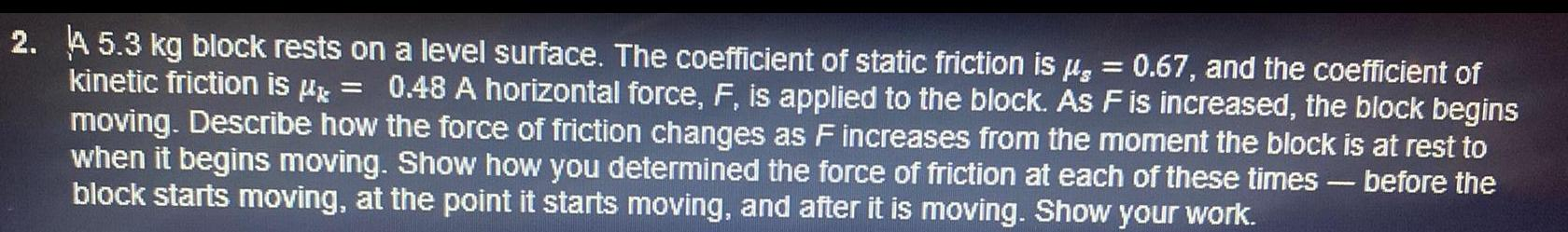Physics
Friction
A 5.3 kg block rests on a level surface. The coefficient of static friction is μ = 0.67, and the coefficient of kinetic friction is μ* = 0.48 A horizontal force, F, is applied to the block. As F is increased, the block begins moving. Describe how the force of friction changes as F increases from the moment the block is at rest to when it begins moving. Show how you determined the force of friction at each of these times - before the block starts moving, at the point it starts moving, and after it is moving. Show your work.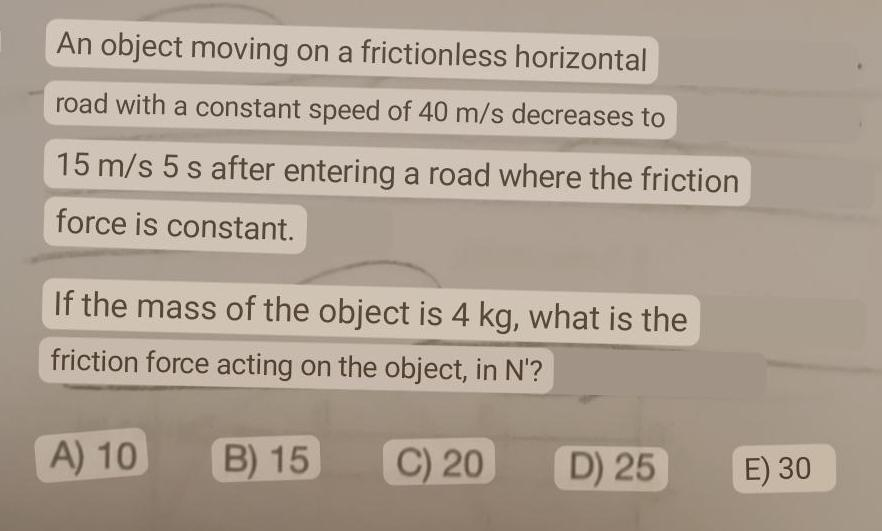Physics
Friction
An object moving on a frictionless horizontal road with a constant speed of 40 m/s decreases to 15 m/s 5 s after entering a road where the friction force is constant. If the mass of the object is 4 kg, what is the friction force acting on the object, in N'? A) 10 B) 15 C) 20 D) 25 E) 30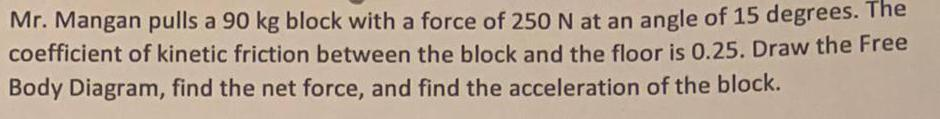Physics
Friction
Mr. Mangan pulls a 90 kg block with a force of 250 N at an angle of 15 degrees. The coefficient of kinetic friction between the block and the floor is 0.25. Draw the Free Body Diagram, find the net force, and find the acceleration of the block.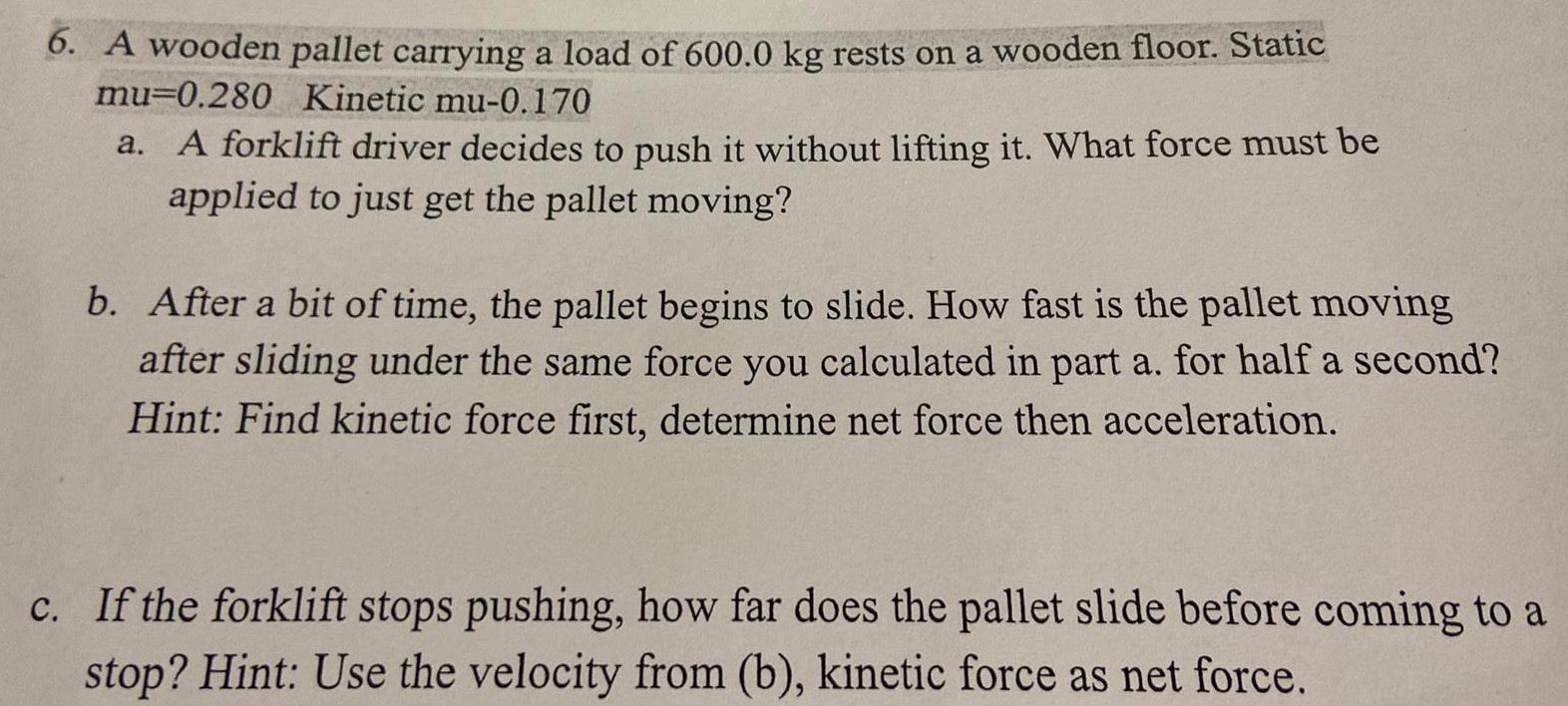Physics
Friction
6. A wooden pallet carrying a load of 600.0 kg rests on a wooden floor. Static mu-0.280 Kinetic mu-0.170 a. A forklift driver decides to push it without lifting it. What force must be applied to just get the pallet moving? b. After a bit of time, the pallet begins to slide. How fast is the pallet moving after sliding under the same force you calculated in part a. for half a second? Hint: Find kinetic force first, determine net force then acceleration. c. If the forklift stops pushing, how far does the pallet slide before coming to a stop? Hint: Use the velocity from (b), kinetic force as net force.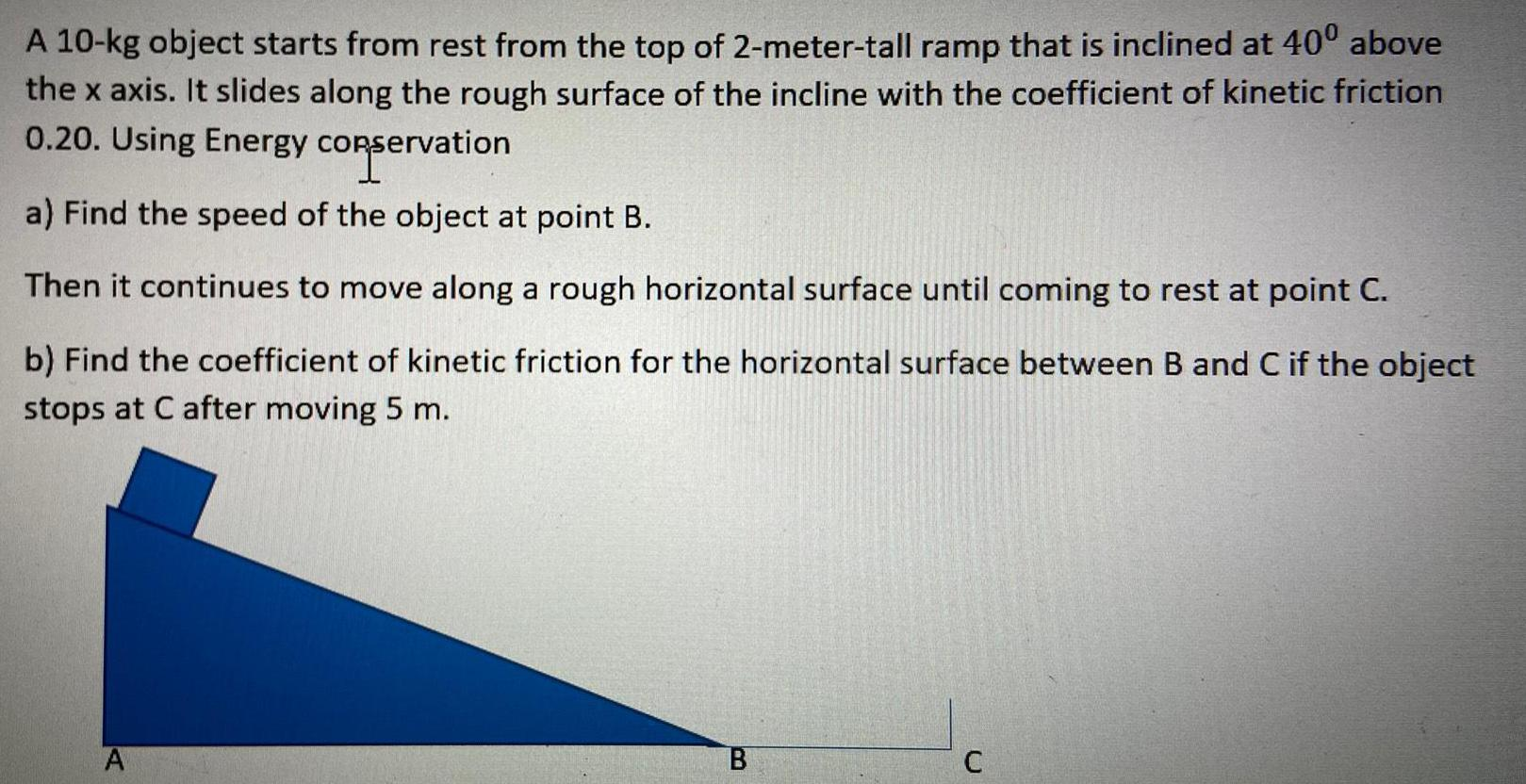Physics
Friction
A 10-kg object starts from rest from the top of 2-meter-tall ramp that is inclined at 40⁰ above the x axis. It slides along the rough surface of the incline with the coefficient of kinetic friction 0.20. Using Energy conservati vation a) Find the speed of the object at point B. Then it continues to move along a rough horizontal surface until coming to rest at point C. b) Find the coefficient of kinetic friction for the horizontal surface between B and C if the object stops at C after moving 5 m.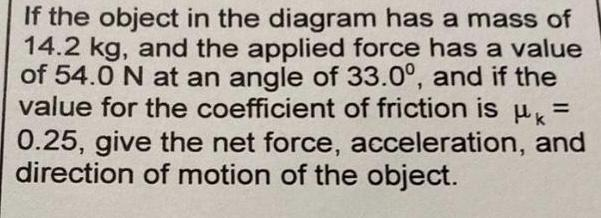Physics
Friction
If the object in the diagram has a mass of 14.2 kg, and the applied force has a value of 54.0 N at an angle of 33.0°, and if the value for the coefficient of friction is μ = 0.25, give the net force, acceleration, and direction of motion of the object.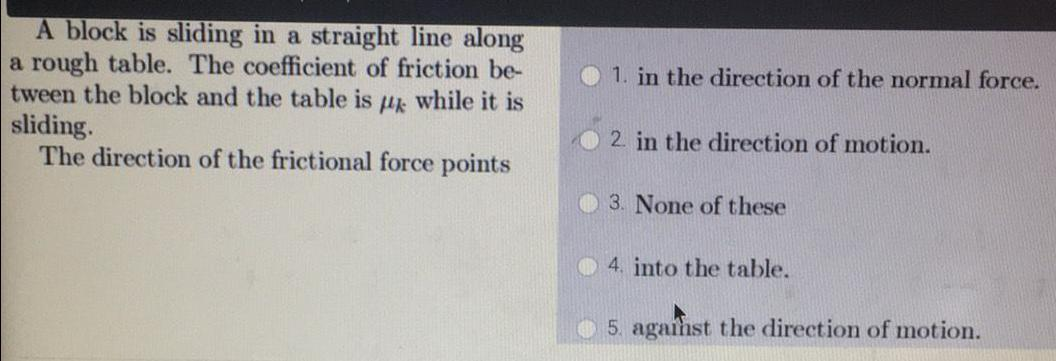Physics
Friction
A block is sliding in a straight line along a rough table. The coefficient of friction be- tween the block and the table is mk while it is sliding. The direction of the frictional force points 1. in the direction of the normal force. 2. in the direction of motion. 3. None of these 4. into the table. 5. against the direction of motion.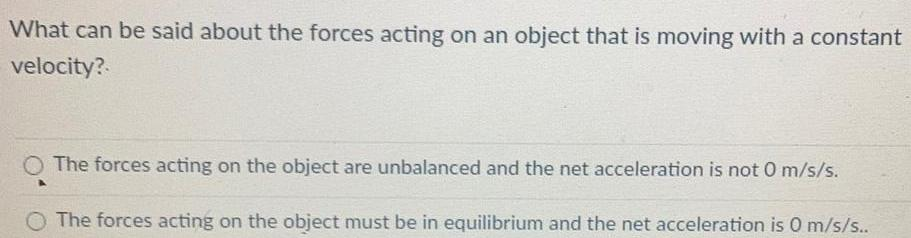Physics
Friction
What can be said about the forces acting on an object that is moving with a constant velocity? The forces acting on the object are unbalanced and the net acceleration is not 0 m/s/s. The forces acting on the object must be in equilibrium and the net acceleration is 0 m/s/s..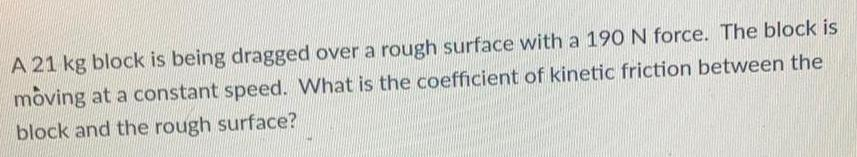Physics
Friction
A 21 kg block is being dragged over a rough surface with a 190 N force. The block is moving at a constant speed. What is the coefficient of kinetic friction between the block and the rough surface?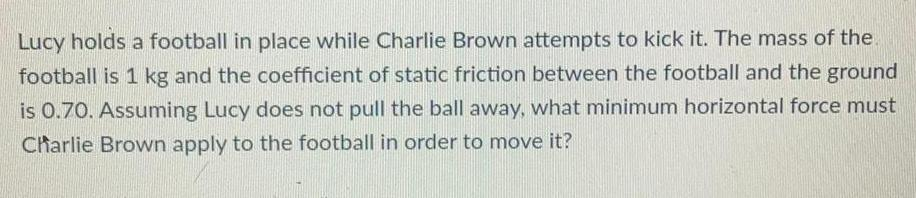Physics
Friction
Lucy holds a football in place while Charlie Brown attempts to kick it. The mass of the. football is 1 kg and the coefficient of static friction between the football and the ground is 0.70. Assuming Lucy does not pull the ball away, what minimum horizontal force must Charlie Brown apply to the football in order to move it?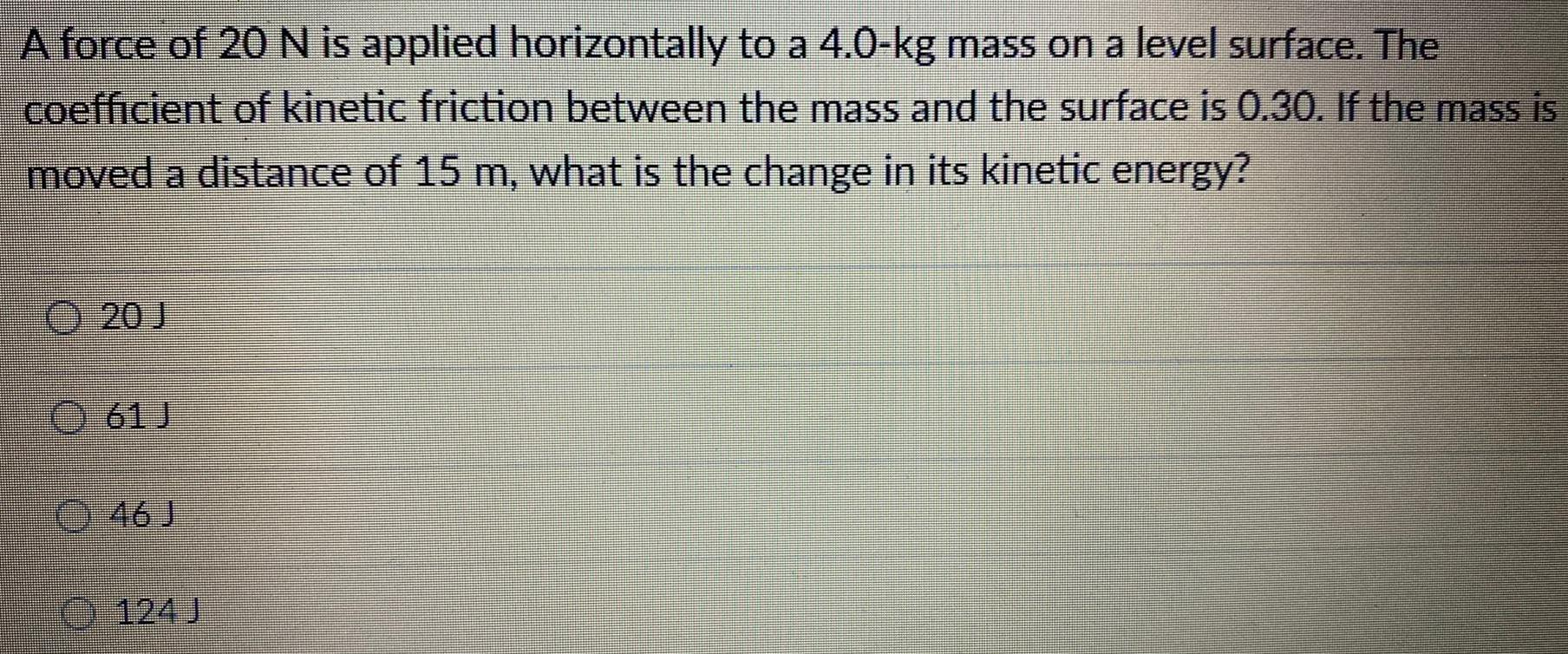Physics
Friction
A force of 20 N is applied horizontally to a 4.0-kg mass on a level surface. The coefficient of kinetic friction between the mass and the surface is 0.30. If the mass is moved a distance of 15 m, what is the change in its kinetic energy? 20 J 61J 46J 124J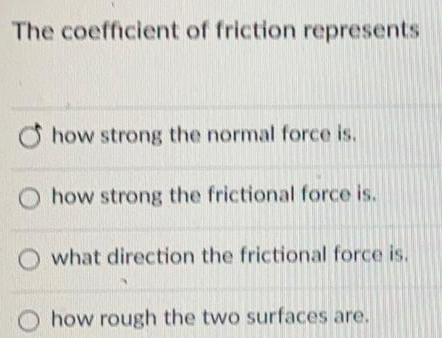Physics
Friction
The coefficient of friction represents how strong the normal force is. how strong the frictional force is. what direction the frictional force is. Ohow rough the two surfaces are.